# Capacitive Transducer

Definition: The capacitive transducer is used for measuring the displacement, pressure and other physical quantities. It is a passive transducer that means it requires external power for operation. The capacitive transducer works on the principle of variable capacitances. The capacitance of the capacitive transducer changes because of many reasons like overlapping of plates, change in distance between the plates and dielectric constant.

The capacitive transducer contains two parallel metal plates. These plates are separated by the dielectric medium which is either air, material, gas or liquid. In the normal capacitor the distance between the plates are fixed, but in capacitive transducer the distance between them are varied.

The capacitive transducer uses the electrical quantity of capacitance for converting the mechanical movement into an electrical signal. The input quantity causes the change of the capacitance which is directly measured by the capacitive transducer.

The capacitors measure both the static and dynamic changes. The displacement is also measured directly by connecting the measurable devices to the movable plate of the capacitor. It works on with both the contacting and non-contacting modes.

## Principle of Operation

The equations below express the capacitance between the plates of a capacitor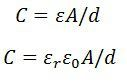Where A – overlapping area of plates in m2
d – the distance between two plates in meter
ε – permittivity of the medium in F/m
εr – relative permittivity
ε0 – the permittivity of free space

The schematic diagram of a parallel plate capacitive transducer is shown in the figure below.The change in capacitance occurs because of the physicals variables like displacement, force, pressure, etc. The capacitance of the transducer also changes by the variation in their dielectric constant which is usually because of the measurement of liquid or gas level.

The capacitance of the transducer is measured with the bridge circuit. The output impedance of transducer is given asWhere, C – capacitance
f – frequency of excitation in Hz.

The capacitive transducer is mainly used for measurement of linear displacement. The capacitive transducer uses the following three effects.

1. Variation in capacitance of transducer is because of the overlapping of capacitor plates.
2. The change in capacitance is because of the change in distances between the plates.
3. The capacitance changes because of dielectric constant.

The following methods are used for the measuring displacement.

1. A transducer using the change in the Area of Plates – The equation below shows that the capacitance is directly proportional to the area of the plates. The capacitance changes correspondingly with the change in the position of the plates.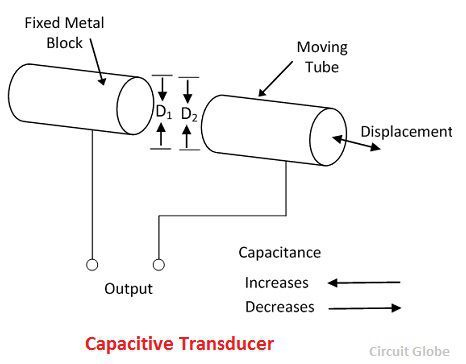The capacitive transducers are used for measuring the large displacement approximately from 1mm to several cms. The area of the capacitive transducer changes linearly with the capacitance and the displacement. Initially, the nonlinearity occurs in the system because of the edges. Otherwise, it gives the linear response.

The capacitance of the parallel plates is given aswhere x – the length of overlapping part of plates
ω – the width of overlapping part of plates.

The sensitivity of the displacement is constant, and therefore it gives the linear relation between the capacitance and displacement.The capacitive transducer is used for measuring the angular displacement. It is measured by the movable plates shown below. One of the plates of the transducer is fixed, and the other is movable.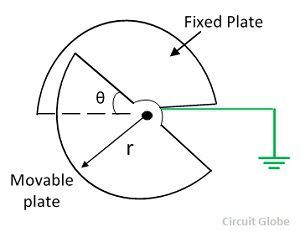The phasor diagram of the transducer is shown in the figure below.The angular movement changes the capacitance of the transducers. The capacitance between them is maximum when these plates overlap each other. The maximum value of capacitance is expressed asThe capacitance at angle θ is given expressed as,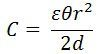θ – angular displacement in radian. The sensitivity for the change in capacitance is given as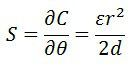The 180° is the maximum value of the angular displacement of the capacitor.

2. The transducer using the change in distance between the plates – The capacitance of the transducer is inversely proportional to the distance between the plates. The one plate of the transducer is fixed, and the other is movable. The displacement which is to be measured links to the movable plates.The capacitance is inversely proportional to the distance because of which the capacitor shows the nonlinear response. Such type of transducer is used for measuring the small displacement. The phasor diagram of the capacitor is shown in the figure below.The sensitivity of the transducer is not constant and vary from places to places.

The following are the major advantages of capacitive transducers.

1. It requires an external force for operation and hence very useful for small systems.
2. The capacitive transducer is very sensitive.
3. It gives good frequency response because of which it is used for the dynamic study.
4. The transducer has high input impedance hence they have a small loading effect.
5. It requires small output power for operation.

The main disadvantages of the transducer are as follows.

1. The metallic parts of the transducers require insulation.
2. The frame of the capacitor requires earthing for reducing the effect of the stray magnetic field.
3. Sometimes the transducer shows the nonlinear behaviours because of the edge effect which is controlled by using the guard ring.
4. The cable connecting across the transducer causes an error.

### Uses of Capacitive Transducer

The following are the uses of capacitive transducer.

1. The capacitive transducer uses for measurement of both the linear and angular displacement. It is extremely sensitive and used for the measurement of very small distance.
2. It is used for the measurement of the force and pressures. The force or pressure, which is to be measured is first converted into a displacement, and then the displacement changes the capacitances of the transducer.
3. It is used as a pressure transducer in some cases, where the dielectric constant of the transducer changes with the pressure.
4. The humidity in gases is measured through the capacitive transducer.
5. The transducer uses the mechanical modifier for measuring the volume, density, weight etc.

The accuracy of the transducer depends on the variation of temperature to the high level.

### 7 thoughts on “Capacitive Transducer”

1. I need to know how the capacitance in level measurement is used to determine the height of the substance in a closed container .